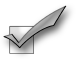### Home > GB8I > Chapter 6 Unit 7 > Lesson INT1: 6.3.3 > Problem6-111

6-111.This problem is the checkpoint for solving linear equations (with fractional coefficients). It will be referred to as Checkpoint 6A.

Solve each equation.

1. $\frac { 1 } { 6 }m − 3 = −5$

1. $\frac{2}{3}x-3=\frac { 1 } { 2 }x − 7$

1. $x + \frac { x } { 2 }-4=\frac{x}{4}$

Check your answers by referring to the Checkpoint 6A materials located at the back of your book.

Ideally, at this point you are comfortable working with these types of problems and can solve them correctly. If you feel that you need more confidence when solving these types of problems, then review the Checkpoint 6A materials and try the practice problems provided. From this point on, you will be expected to do problems like these correctly and with confidence.

Answers and extra practice are located in the back of your printed textbook or in the Reference Tab of your eBook. If you have an eBook for INT1, login and then click the following link: